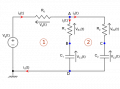# How to solve this circuit?

#### Flagy

Joined Jan 28, 2020
3
Given the attached schema I'd love to find the analytical temporal and frequency expressions for the current in the 2 branches: i1 and i2.
R0, R1, R2, C1, C2 and Vg(t) are given.
In the time domain can you show me how to set the system of differential equations that will bring me to the solution? Once I have the system it shouldn't be a problem to solve it using for instance odeint in Python.
Thank you in advance!#### Dodgydave

Joined Jun 22, 2012
8,825

#### Flagy

Joined Jan 28, 2020
3
Homework is it?
more or less, it's more for work actually. The fact is that my background in electric circuits is not optimal, but I see it may appears as homeworks for experts#### WBahn

Joined Mar 31, 2012
25,249
First, apply KVL and KCL to the circuit and then express each term's voltage/current relationship using the constitutive equation for that component, taking into account the initial conditions.

#### Flagy

Joined Jan 28, 2020
3
First, apply KVL and KCL to the circuit and then express each term's voltage/current relationship using the constitutive equation for that component, taking into account the initial conditions.
Can you show me how?

#### WBahn

Joined Mar 31, 2012
25,249
Can you show me how?
Go get an introductory text on circuit analysis and read how to do it. Then, if you are still having problems, post your best attempt and we can help you figure out where you are going wrong.

#### MrAl

Joined Jun 17, 2014
7,147
Given the attached schema I'd love to find the analytical temporal and frequency expressions for the current in the 2 branches: i1 and i2.
R0, R1, R2, C1, C2 and Vg(t) are given.
In the time domain can you show me how to set the system of differential equations that will bring me to the solution? Once I have the system it shouldn't be a problem to solve it using for instance odeint in Python.
Thank you in advance!View attachment 197729
The key to solving this using differential equations like a set of ODE's is to understand a simple property of capacitors. That is, the way the voltage and current are related in a capacitor.
For a resistor, we know:
i=E/R

and for a cap we have a relationship also but here is where the differential equation comes in:
i=C*d(Vc)/dt

So very simply, the current through the cap is the capacitance value times the first derivative of the capacitor voltage with respect to time. This is what allows you to write the ODE's.

Since we have two capacitors we have two currents and two voltages and two derivatives.
i1=C1*d(Vc1)/dt
i2=C2*d(Vc2)/dt

All what is left to do now is write the two ODE's.
To help with this, try replacing the two capacitors with voltage sources and solve for the current through each voltage source, calling the two voltage sources Vc1 and Vc2 but maintaining that the two currents are still related to the derivatives.

See if that helps. Note that once you assume that you "know" the two voltages of the two caps it gets easier to write the equations.

As a quick example, say we have a single DC voltage source E and single resistor in series with a cap, and the DC voltage source powers the resistor and cap and we want to solve fro the voltage across the cap in time assuming Vc(0)=0 volts.
The equation goes as:
iC=(E-Vc)/R
which simply states that the current through the cap is equal to the source voltage minus the cap voltage divided by the resistor value R.
Well, we know the relationship of the current to the voltage is iC=C*dv/dt so we can substitute that into the previous equation:
C*d(Vc)/dt=(E-Vc)/R

and now to get the ODE we just have to divide by C:
d(Vc)/dt=(E-Vc)/(R*C)

and that is the required ODE.

Now in your circuit, you have two caps and therefore two voltages and also have two currents, but the equations write 'almost' the same although you also have to know that the sum of currents into a node equals zero.

See what you can do with this now.

Last edited:

#### RBR1317

Joined Nov 13, 2010
522
find the analytical temporal and frequency expressions for the current in the 2 branches: i1 and i2.
The assignment is to find the current in the 2 branches using differential equations. So, what if I found the voltage at node A using Laplace transforms? You might be able to use the node A voltage to check the correctness of the ODE current solution.

We know Vg(t) is given but don't know what it is given as; so assume it is the unit step function with magnitude Vg. (This translates to Vg/s in the complex frequency domain.)

The attachment shows the node voltage equation at point A as expressed in the complex frequency domain; solving for the node voltage Va; taking the inverse Laplace transform to get the time domain solution for the voltage Va.